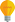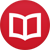# Integral of $\sin^2(x)$

by Jakub MarianTip: See my list of theMost Common Mistakes in English. It will teach you how to avoid mis­takes with com­mas, pre­pos­i­tions, ir­reg­u­lar verbs, and much more.

The integral can be calculated using integration by parts (using the formula $∫u’(x)v(x)\,dx = u(x)v(x) - ∫ u(x)v’(x)\,dx$). Let’s write $\sin^2(x)$ as $\sin(x)\sin(x)$ and apply this formula:

$$∫ \sin(x)\sin(x)\,dx = -\cos(x)\sin(x)-∫(-\cos(x))\cos(x)\,dx$$

If we apply integration by parts to the rightmost expression again, we will get $∫\sin^2(x)dx = ∫\sin^2(x)dx$, which is not very useful. The trick is to rewrite the $\cos^2(x)$ in the second step as $1-\sin^2(x)$. Then we get

\begin{align*} ∫ \sin^2(x)\,dx &= -\cos(x)\sin(x)+∫(1-\sin^2(x))\,dx \\ &= -\cos(x)\sin(x)+x-∫\sin^2(x)\,dx \end{align*}

Now, all we have to do is to transfer $∫\sin^2(x)\,dx$ from the right-hand side to the left-hand side of the equation:

$$2∫ \sin^2(x)\,dx = -\cos(x)\sin(x)+x + C$$ $$∫ \sin^2(x)\,dx = ÷{1}{2}(x-\cos(x)\sin(x))+C$$

(Technically speaking, we should write $C/2$ rather than $C$, but it is still just an indefinite constant, so, as is customary, we rename $C/2$ to $C$ to get the result in the usual form.)

By the way, I have written several educational ebooks. If you get a copy, you can learn new things and support this website at the same time—why don’t you check them out?